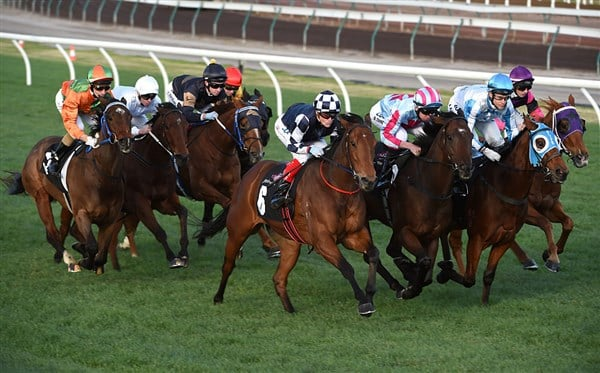# How to calculate a Quaddie percentageOne of the most popular betting types available is the Quaddie, or Quadrella as it is fully known, and we will explain how to calculate a Quaddie percentage.

Before we begin calculating a Quaddie percentage, we will explain what a Quaddie bet actually is and how to successfully land one.

A Quaddie is a bet type where you must successfully predict the winner of four nominated races from a specific meeting. Each race is called a ‘leg’ of the Quaddie and all four races will consecutively follow one another. That is, the Quaddie at a meeting may be Races 4, 5, 6 and 7 or it could be Races 6, 7, 8 and 9 etc.

This sounds difficult to land all four legs, but you can take multiple runners in any leg (in fact, you can take the entire field if you wish).

If you land all four legs, then you have landed the Quaddie and will receive a dividend. The amount that is returned back to you is based upon the Quaddie percentage that you had placed.

HOW TO CALCULATE A QUADDIE PERCENTAGE

You can either use a calculator or your own mind if you’re good with numbers to calculate a Quaddie percentage.

Add up the number of runners that you have chosen for each leg and multiply them together. For example, if you selected three runners in the first leg, four runners in the second leg, two runners in the third leg and six runners in the fourth leg, your calculation would be the following:

3 x 4 x 2 x 6 = 144

This means that it will cost you \$144 if you wish to receive 100% of the Quaddie dividend should you successfully land all four legs of the Quaddie.

Now this amount will sound expensive for the majority of punters, but flexi betting is available for exotics, including Quaddies, so read on to find out how you can spend less to make the Quaddie more affordable for your own budget.

You do not have to take 100% of the Quaddie dividend. Thanks to flexi betting, you can select the amount that you wish to stake and a calculated percentage will be displayed on the screen prior to placing your bet.

Of course we are here to help explain how to calculate a Quaddie percentage; that includes a Flexi Quaddie so here is how you would calculate the Quaddie percentage for the above example.

While \$144 will give you 100% of the Quaddie dividend as outlined above, you may wish to only spend \$50 on the Quaddie. Your calculation would be as follows:

50 / 144 = 0.3472

Multiply this decimal figure by 100 and you get the percentage:

0.3472 x 100 = 34.72%

Therefore, your \$50 stake using the following combination of three, four, two and six runners for the four legs of the Quaddie will return 34.72% of the Quaddie dividend if you are successful.Let’s assume that you land the Quaddie and it returns \$1,273.80. How do you calculate your Quaddie return?